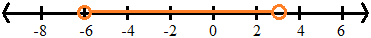Absolute Value

Absolute Value

Absolute value is a number's distance from 0.

|6|  =  6

|-8|  =  8

|0|  =  0

When solving equations or inequalities involving absolute value, it may be helpful to translate the expression into English in order to understand what the expression means.

|x| = 5 states that x is exactly 5 units away from zero.

• This expression may be written x = 5 or x = -5.

lxl < 5  states that x is less than 5 units away from zero.

• Either x is greater than -5 or less than +5.
• This expression may be written -5 < x < 5.

lxl > 5  states that x is more than 5 units away from zero.

• Either x is greater than 5 or x is less than -5.
• This expression may be written x > 5 or x < -5.

EXAMPLE 1

Solve the equation |3x - 5| = 13.

You can re-express the equation as 3x - 5 = 13 or 3x - 5 = -13.

Solving these equations separately:

3x - 5 + 5 = 13 + 5          3x - 5 + 5 = -13 + 5

3x = 18                            3x = -8

3x / 3 = 18 / 3                  3x / 3 = -8 / 3

x = 6                                x = -8/3

The solution to the equation is the set x = {-8/3, 6}.

EXAMPLE 2

Solve and graph the inequality |2x + 3| < 9.

Re-expressing the inequality as -9 < 2x + 3 < 9, we can solve this by operating on all three sides:

-9 - 3 < 2x + 3 - 3 < 9 - 3

-12 < 2x < 6

-12 / 2 < 2x / 2 < 6 / 2

-6 < x < 3

The solution is the interval (-6, 3), and is graphed on the number line as.

Remember... whenever you divide an inequality by a negative number, the symbol(s) change direction.